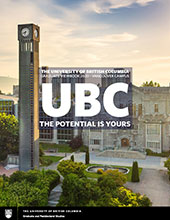# Differential equations and integral equations in pure mathematics

This subclass comprises research and experimental development in differential equations and integral equations in pure mathematics.

#### UBC Researchers conducting research in Differential equations and integral equations in pure mathematics

##### French Name
Équations différentielles et équations intégrales en mathématiques pures
##### French Description
Cette sous-classe comprend la recherche et le développement expérimental avec l'analyse classique en mathématiques pures.